# MCQ Questions for Class 8 Maths Chapter 5 Data Handling with Answers

Check the below NCERT MCQ Questions for Class 8 Maths Chapter 5 Data Handling with Answers Pdf free download. MCQ Questions for Class 8 Maths with Answers were prepared based on the latest exam pattern. We have provided Data Handling Class 8 Maths MCQs Questions with Answers to help students understand the concept very well. https://mcqquestions.guru/mcq-questions-for-class-8-maths-chapter-5/

Students can also refer to NCERT Solutions for Class 8 Maths Chapter 5 Data Handling for better exam preparation and score more marks.

## Data Handling Class 8 MCQs Questions with Answers

I. Observe the following bar graph carefully and answer the following questions :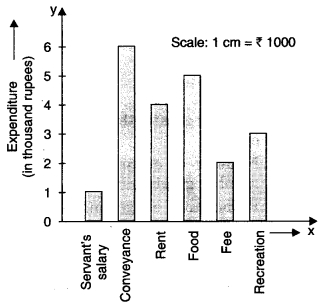Class 8 Maths Chapter 5 MCQ Question 1.
On which item has the maximum expenditure been done ?
(a) Conveyance
(b) Rent
(c) Fee
(d) Servant’s salary.

Hint:
The height of the bar corresponding to conveyance is the maximum.

Data Handling Class 8 MCQ Questions Question 2.
On which item has the minimum expenditure been done ?
(a) Servant’s salary
(b) Food
(c) Rent
(d) Conveyance.

Hint:
The height of the bar corresponding to servant’s salary is minimum.

Data Handling Class 8 MCQ Question 3.
What is the expenditure done on food ?
(a) Rs 1000
(b) Rs 2000
(c) Rs 3000
(d) Rs 5000.

Hint:
Expenditure done on food = 5 × 1000
= Rs 5000.

MCQ On Data Handling For Class 8 Question 4.
What is the difference of expenditures done on conveyance and rent ?
(a) Rs 1000
(b) Rs 2000
(c) Rs 3000
(d) Rs 4000.

Hint:
Expenditure done on conveyance
= 6 × 1000 = Rs 6000
Expenditure done on rent = 4 × 1000
= Rs 4000
∴ Difference = Rs 6000 – Rs 4000
= Rs 2000.

MCQ Questions For Class 8 Maths Chapter 5 Question 5.
Rs 5000 is the expenditure done on
(a) rent
(b) food
(c) fee
(d) recreation.

Hint:
$$\frac{5000}{1000}$$ = 5 cm is the height of the bar corresponding to food.

Class 8 Maths Ch 5 MCQ Question 6.
Rs 6000 is the expenditure done on
(a) fee
(b) rent
(c) conveyance
(d) food.

Hint:
$$\frac{6000}{1000}$$ = 6 cm is the height of the bar corresponding to conveyance.

MCQ Of Data Handling Class 8 Question 7.
How much expenditure has been done in all ?
(a) Rs 21000
(b) Rs 18000
(c) Rs 15000
(d) Rs 20000.

Hint:
Total expenditure
= (1 + 6 + 4 + 5 + 2 + 3) × 1000 = Rs 21000

II. Observe the following bar graph carefully and answer the following questions :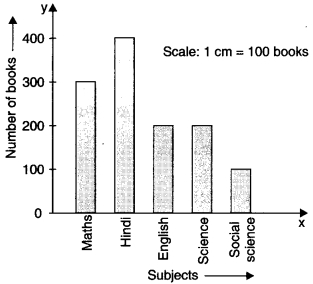Data Handling MCQ Class 8 Question 8.
Of which subject are there the maximum books ?
(a) Hindi
(b) English
(c) Maths
(d) Science.

Hint:
The height of the bar corresponding to Hindi is maximum.

Ncert Class 8 Maths Chapter 5 MCQ Questions Question 9.
How many books are there of the subject whose books are maximum ?
(a) 100
(b) 200
(c) 300
(d) 400.

Hint:
Hindi → 400.

Ch 5 Maths Class 8 MCQ Question 10.
Of which subject are there the minimum books ?
(a) Social Science
(b) Hindi
(c) English
(d) Science.

Hint:
The height of the bar corresponding to Social Science is minimum.

Class 8 Data Handling MCQ Question 11.
How many books are there of the subject whose books are minimum ?
(a) 100
(b) 200
(c) 300
(d) 400.

Hint:
Social Science → 100.

Class 8 Chapter 5 Maths MCQ Question 12.
Which two subjects have the same number of books ?
(a) Maths and Hindi
(b) Hindi and English
(c) English and Science
(d) Science and Social Science.

Hint:
English → 200
Science → 200

MCQ Class 8 Maths Chapter 5 Question 13.
300 books are of the subject
(a) Maths
(b) English
(c) Hindi
(d) Science.

Hint:
300 → Maths.

Class 8 Math Chapter 5 MCQ Question 14.
The difference of the number of books of English and Science is
(a) 200
(b) 100
(c) 400
(d) 0.

Hint:
200 – 200 = 0.

Question 15.
The difference of the number of books of Hindi and Social Science is
(a) 200
(b) 300
(c) 400
(d) 100.

Hint:
400 – 100 = 300.

Question 16.
The total number of books is
(a) 1200
(b) 1400
(c) 1600
(d) 1800.

Hint:
300 + 400 + 200 + 200 + 100 = 1200.

Question 17.
The total of the number of books of English and Science is
(a) 200
(b) 100
(c) 400
(d) 0.

Hint:
200 + 200 = 400.

III. Study the following frequency distribution table and answer the questions given below :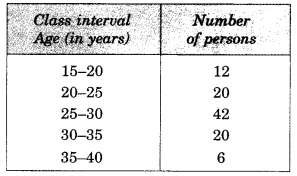Question 18.
What is the size of the class intervals ?
(a) 5
(b) 10
(c) 15
(d) 20.

Hint:
Size = 20 – 15 = 5.

Question 19.
Which class has the highest frequency ?
(a) 15-20
(b)20-25
(c) 25-30
(d) 35-40.

Hint:
Highest frequency = 42 → 25 – 30.

Question 20.
Which class has the lowest frequency ?
(a) 35-40
(b)30-35
(c) 15-20
(d) 25-30.

Hint:
Lowest frequency = 6 → 35 – 40.

Question 21.
Which two classes have the same frequency ?
(а) 15-20 and 35-40
(b) 20-25 and 30-35
(c) 15-20 and 30-35
(d) 20-25 and 25-30.

Hint:
Frequency of class 20-25 = Frequency of class 30-35 = 20.

Question 22.
What is the upper limit of the class interval 25-30 ?
(a) 20
(b)25
(c) 30
(d) 35.

Question 23.
What is the lower limit of the class interval 35-40 ?
(a) 20
(b) 25
(c) 30
(d) 35.

Question 24.
The difference between the frequencies of the class intervals 20-25 and 30-35 is
(a) 0
(b) 10
(c) 20
(d) 5.

Hint:
Frequency of class 20-25 = 20.
Frequency of class 30-35 = 20
∴ Difference = 20-20 = 0.

IV. Study the following frequency distribution table and answer the questions given below :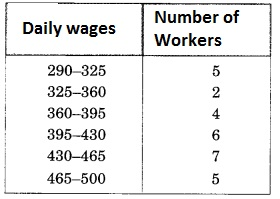Question 25.
The upper limit of the fourth class is
(a) 430
(b) 395
(c) 465
(d) 500.

Hint:
Size = 325 – 290 = 35.

Question 26.
The lower limit of the sixth class is
(a) 395
(b) 430
(c) 465
(d) 360.

Hint:
Sixth class is 465 – 500.

Question 27.
The size of the class intervals is
(a) 25
(b) 30
(c) 40
(d) 35.

Hint:
Fourth class is 395-430.

Question 28.
The frequency of the third class is
(a) 5
(b) 4
(c) 2
(d) 7.

Hint:
Third class is 360 – 395.

Question 29.
The number of workers getting daily wages 395-430 (in ?) is
(a) 4
(b) 5
(c) 6
(d) 7.

Hint:
Frequency of class 395 – 430 is 6.

Question 30.
Which two classes have the same frequency ?
(a) 290-325 and 465-500
(b) 290-325 and 325-360
(c) 430-465 and 465-500
(d) 325-360 and 360-395.

Hint:
Frequency of class 290-325
= Frequency of class 465-500 = 5.

Question 31.
The class with highest frequency is
(a) 430-465
(b) 465-500
(c) 395-430
(d) 290-325.

Hint:
Highest frequency = 7 → 430-465.

Question 32.
The class with lowest frequency is
(a) 325-360
(b) 360-395
(c) 465-500
(d) 395-430.

Hint:
Lowest frequency = 2 → 325-360.

Question 33.
The number of workers getting wages ? 395 and above is
(a) 18
(b) 24
(c) 12
(d) 28.

Hint:
Required number = 6 + 7 + 5 = 18.

Question 34.
The number of workers getting wages below ? 360 is
(a) 7
(b) 6
(c) 5
(d) 4.

Hint:
Required number = 5 + 2 = 7.

Question 35.
The total number of workers is
(a) 29
(b) 22
(c) 28
(d) 21.

Hint:
Required number = 5 + 2 + 4 + 6 + 7 + 5
= 29.

V. Observe the histogram and answer the questions given below :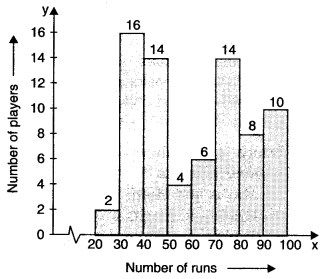Question 36.
Which group contains maximum players ?
(a) 20-30
(b) 30-40
(c) 40-50
(d) 70-80.

Hint:
Maximum frequency = 16 → 30-40.

Question 37.
Which group has minimum players ?
(a) 20-30
(b) 50-60
(c) 60-70
(d) 30-40.

Hint:
Minimum frequency = 2 → 20-30.

Question 38.
Which two groups have the same number of players ?
(a) 30-40 and 40-50
(b) 20-30 and 30-40
(c) 40-50 and 70-80
(d) 80-90 and 90-100.

Hint:
Frequency of group 40-50 = 14
= Frequency of group 70-80.

Question 39.
How many players make runs 80 and above ?
(a) 2
(b) 8
(c) 10
(d) 18.

Hint:
Required number = 8 + 10 = 18.

Question 40.
How many players make runs less than 40?
(a) 8
(b) 2
(c) 18
(d) 10.

Hint:
Required number = 2 + 16 = 18.

Question 41.
How many players make runs 50 to less than 60 ?
(a) 1
(b) 2
(c) 3
(d) 4

Hint:
Required number
= Frequency of group 50-60 = 4.

Question 42.
The total number of players making runs 20 and more is
(a) 74
(b) 64
(c) 84
(d) 54.

Hint:
Required number
= 2 + 16 + 14 + 4 + 6 + 14 + 8 + 10 = 74.

VI. Observe the histogram and answer the questions given below :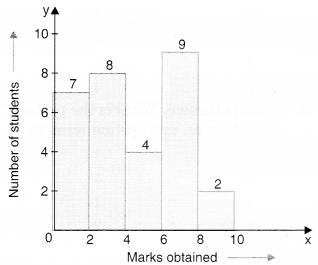Question 43.
The total number of students is
(a) 10
(b) 20
(c) 25
(d) 30.

Hint:
Total number of students
= 7 + 8 + 4 + 9 + 2 = 30.

Question 44.
Which groups contain the maximum number of students ?
(a) 2-4
(b) 4-6
(c) 6-8
(d) 8-10.

Hint:
Maximum number of students
= 9 → 6 – 8.

Question 45.
Which group contains the minimum number of students ?
(a) 0-2
(b) 2-4
(c) 6-8
(d) 8-10.

Hint:
Minimum number of students
= 2 → 8 -10.

Question 46.
The number of students getting marks 6 and above is
(a) 11
(b) 10
(c) 12
(d) 9.

Hint:
Required number = 9 + 2 = 11.

Question 47.
The number of students getting marks 4 to less than 6 is
(a) 2
(b) 4
(c) 6
(d) 8.

Hint:
Required number
= Frequency of class (4 – 6) = 4.

Question 48.
The number of students getting marks less than 4 is
(a) 10
(b) 15
(c) 7
(d) 8

Hint:
Required number = 7 + 8 = 15.

Question 49.
The number of students getting marks in the groups 4-6 or 8-10 is
(a) 2
(b) 4
(c) 6
(d) 8.

Hint:
Required number = 4 + 2 = 6.

Question 50.
The number of students getting marks 6-8 is greater than the number of students getting marks 2-4 by
(a) 1
(b) 2
(c) 3
(d) 4.

Hint:
Required difference = 9 – 8 = 1.

VII. Observe the pie chart given below and answer the following questions: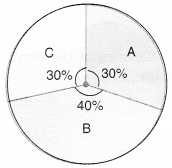Question 51.
The central angle for sector A is
(a) 108°
(b) 144°
(c) 72°
(d) 150°.

Hint:
Central angle for sector A
= $$\frac{30}{100}$$ × 360° = 108°.

Question 52.
The central angle for sector B is
(a) 108°
(b) 144°
(c) 72°
(d) 120°.

Hint:
Central angle for sector B
= $$\frac{40}{100}$$ × 360° = 144°.

Question 53.
Which sector has the greatest angle ?
(a) A
(b) B
(c) C
(d) None of these.

Hint:
Greatest percentage = 40% → B.

Question 54.
What is the difference between the central angles for sector B and sector C ?
(a) 36°
(b) 72°
(c) 9°
(d) 81°.

Hint:
144° – 108° = 36°.

VII. Observe the pie chart and answer the following questions :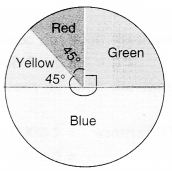Question 55.
Which two colours have the same central angles ?
(a) Red, yellow
(b) Red, green
(c) Yellow, green
(d) Blue, red.

Hint:
Red → 45%
Yellow → 45%.

Question 56.
Which colour has the greatest central angle ?
(a) Red
(b) Yellow
(c) Green
(d)Blue.

Hint:
Blue → 180°.

Question 57.
The proportion of sector for red is
(a) $$\frac{1}{2}$$
(b) $$\frac{1}{4}$$
(c) $$\frac{1}{8}$$
(d) $$\frac{1}{3}$$

Hint:
$$\frac{45°}{360°}$$ = $$\frac{1}{8}$$

Question 58.
The difference of the central angles for green and blue is
(a) 45°
(b) 90°
(c) 180°
(d) 22$$\frac{1°}{2}$$

Hint:
Central angle for blue = 180°
Central angle for green = 90°
∴ Difference = 180° – 90° = 90°.

Question 59.
A child has a block in the shape of a cube with one letter written on each face as shown below: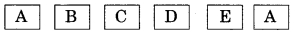The cube is thrown once. What is the probability of getting A ?
(a) $$\frac{1}{3}$$
(b) $$\frac{1}{6}$$
(c) $$\frac{1}{2}$$
(d) $$\frac{1}{4}$$

Hint:
Probability = $$\frac{2}{6}$$ = $$\frac{1}{3}$$

Question 60.
A die is thrown. What is the probability of getting an even prime number ?
(a) $$\frac{1}{6}$$
(b) $$\frac{1}{4}$$
(c) $$\frac{1}{3}$$
(d) $$\frac{1}{2}$$

∴ Probability = $$\frac{1}{6}$$